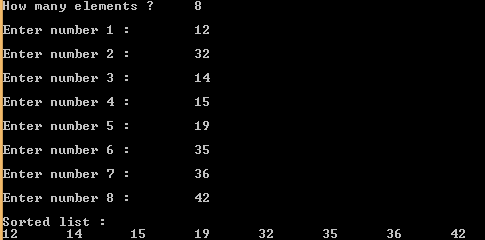## Sunday, 28 December 2014

### C program for sorting elements using bubble sort

 This is a C program that performs sorting of elements using bubble sort. Bubble sort is a technique where each element is compared with its adjacent element , if its adjacent element is smaller then swapping occurs, this continues until every element is checked. See the animation below to understand,

"Bubble-sort-example" by Swfung8 - Own work. Licensed under CC BY-SA 3.0 via Wikimedia Commons.

PROGRAM :
```#include <stdio.h>
#include <stdlib.h>

int main()
{
int n, i, j, swap;
printf("How many elements ?");
scanf("%d", &n);
int array[n];
for (i = 0; i < n; i++)
{
printf("Enter number %d\t", i+1);
scanf("%d", &array[i]);
}

for (i = 0 ; i < ( n - 1 ); i++)
{
for (j = 0 ; j < n - i - 1; j++)
{
if (array[j] > array[j+1])
{
swap       = array[j];
array[j]   = array[j+1];
array[j+1] = swap;
}
}
}
printf("\nSorted list :\n");
for ( i = 0 ; i < n ; i++ )
printf("%d\t", array[i]);
return 0;

}
```

OUTPUT :C - Bubble Sort